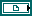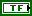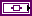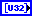# WA Configure Scalogram Indicator VI

LabVIEW 2014 Advanced Signal Processing Toolkit Help

Edition Date: June 2014

Part Number: 372656C-01

»View Product InfoDownload Help (Windows Only)

Owning Palette: Utilities VIs

Displays the square magnitude or the phase of the wavelet coefficients on an intensity graph. This VI displays the square magnitude of the real-valued wavelet coefficients from the WA Continuous Wavelet Transform VI and displays the square magnitude or the phase of the complex-valued wavelet coefficients from the WA Analytic Wavelet Transform VI. Wire data to the CWT coef input or the AWT coef input to determine the polymorphic instance to use or manually select the instance.

Example

Use the pull-down menu to select an instance of this VI.

 Select an instance WA Configure Scalogram Indicator (Predefined Color Table, Real)WA Configure Scalogram Indicator (Customized Color Table, Real)WA Configure Scalogram Indicator (Predefined Color Table, Complex)WA Configure Scalogram Indicator (Customized Color Table, Complex)

## WA Configure Scalogram Indicator (Predefined Color Table, Real)reference of scalogram indicator specifies the reference of scalogram indicator. If you do not wire this input, this VI does not set the scale information and color table for scalogram indicator.dB on? specifies to display the scalogram in decibels or in a linear scale. If dB on? is TRUE, this VI returns the scalogram in decibels. If dB on? is FALSE, this VI returns the scalogram in a linear scale. The default is FALSE.CWT coef specifies the results of the continuous wavelet transform (CWT).scale info specifies the time information and the scale (frequency) information of CWT coef.ignore timestamp? specifies whether this VI forces the timestamp of the input signal to 0 so you can define the limit signals relative to the beginning of the input signal. The default is FALSE, which means this VI uses the timestamp in scale info.error in describes error conditions that occur before this node runs. This input provides standard error in functionality.color table specifies the color table of scalogram indicator.

 0 Rainbow (default)—Displays the maximum in red and the minimum in purple. 1 Inverse gray—Displays the maximum in black and the minimum in white. 2 Gray—Displays the maximum in white and the minimum in black.scalogram indicator displays the scalogram on an intensity graph. The x-axis represents time, and the y-axis represents the scale, or the frequency. The color indicates the square magnitude or the phase of the signal.error out contains error information. This output provides standard error out functionality.

## WA Configure Scalogram Indicator (Customized Color Table, Real)reference of scalogram indicator specifies the reference of scalogram indicator. If you do not wire this input, this VI does not set the scale information and color table for scalogram indicator.dB on? specifies to display the scalogram in decibels or in a linear scale. If dB on? is TRUE, this VI returns the scalogram in decibels. If dB on? is FALSE, this VI returns the scalogram in a linear scale. The default is FALSE.CWT coef specifies the results of the continuous wavelet transform (CWT).scale info specifies the time information and the scale (frequency) information of CWT coef.ignore timestamp? specifies whether this VI forces the timestamp of the input signal to 0 so you can define the limit signals relative to the beginning of the input signal. The default is FALSE, which means this VI uses the timestamp in scale info.error in describes error conditions that occur before this node runs. This input provides standard error in functionality.color table specifies a 256-element array of colors that defines the color table of scalogram indicator. Refer to the Create IntGraph Color Table VI in the labview\examples\general\graphs\intgraph.llb for an example of how to create a customized color table.scalogram indicator displays the scalogram on an intensity graph. The x-axis represents time, and the y-axis represents the scale, or the frequency. The color indicates the square magnitude or the phase of the signal.error out contains error information. This output provides standard error out functionality.

## WA Configure Scalogram Indicator (Predefined Color Table, Complex)reference of scalogram indicator specifies the reference of scalogram indicator. If you do not wire this input, this VI does not set the scale information and color table for scalogram indicator.dB on? specifies to display the scalogram in decibels or in a linear scale. If dB on? is TRUE, this VI returns the scalogram in decibels. If dB on? is FALSE, this VI returns the scalogram in a linear scale. The default is FALSE.AWT coef specifies the results of the analytic wavelet transform (AWT).scale info specifies the time information and the scale (frequency) information of AWT coef.ignore timestamp? specifies whether this VI forces the timestamp of the input signal to 0 so you can define the limit signals relative to the beginning of the input signal. The default is FALSE, which means this VI uses the timestamp in scale info.error in describes error conditions that occur before this node runs. This input provides standard error in functionality.color table specifies the color table of scalogram indicator.

 0 Rainbow (default)—Displays the maximum in red and the minimum in purple. 1 Inverse gray—Displays the maximum in black and the minimum in white. 2 Gray—Displays the maximum in white and the minimum in black.display type specifies to display the square magnitude or the phase of the coefficients.

 0 Square magnitude (default)—Displays the square magnitude of the coefficients. 1 Phase—Displays the phase of the coefficients.scalogram indicator displays the scalogram on an intensity graph. The x-axis represents time, and the y-axis represents the scale, or the frequency. The color indicates the square magnitude or the phase of the signal.error out contains error information. This output provides standard error out functionality.

## WA Configure Scalogram Indicator (Customized Color Table, Complex)reference of scalogram indicator specifies the reference of scalogram indicator. If you do not wire this input, this VI does not set the scale information and color table for scalogram indicator.dB on? specifies to display the scalogram in decibels or in a linear scale. If dB on? is TRUE, this VI returns the scalogram in decibels. If dB on? is FALSE, this VI returns the scalogram in a linear scale. The default is FALSE.AWT coef specifies the results of the analytic wavelet transform (AWT).scale info specifies the time information and the scale (frequency) information of AWT coef.ignore timestamp? specifies whether this VI forces the timestamp of the input signal to 0 so you can define the limit signals relative to the beginning of the input signal. The default is FALSE, which means this VI uses the timestamp in scale info.error in describes error conditions that occur before this node runs. This input provides standard error in functionality.color table specifies a 256-element array of colors that defines the color table of scalogram indicator. Refer to the Create IntGraph Color Table VI in the labview\examples\general\graphs\intgraph.llb for an example of how to create a customized color table.display type specifies to display the square magnitude or the phase of the coefficients.

 0 Square magnitude (default)—Displays the square magnitude of the coefficients. 1 Phase—Displays the phase of the coefficients.scalogram indicator displays the scalogram on an intensity graph. The x-axis represents time, and the y-axis represents the scale, or the frequency. The color indicates the square magnitude or the phase of the signal.error out contains error information. This output provides standard error out functionality.

## Example

Refer to the Color Tables for Displaying the Scalogram VI in the labview\examples\Wavelet Analysis\WAGettingStarted directory for an example of using the WA Configure Scalogram Indicator VI.